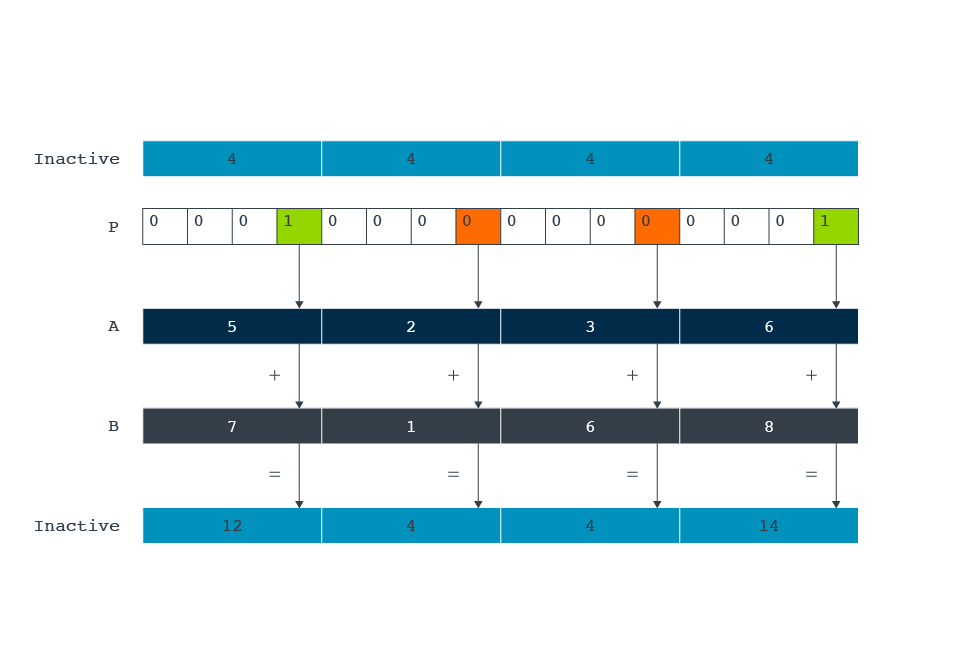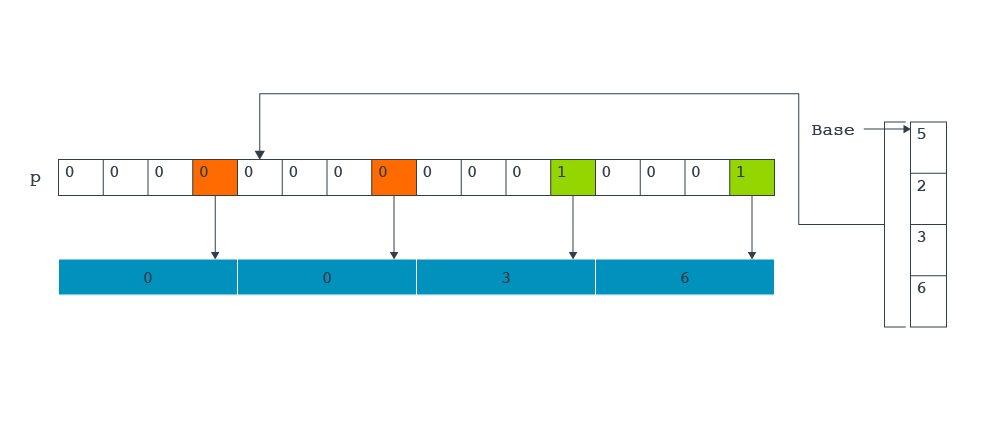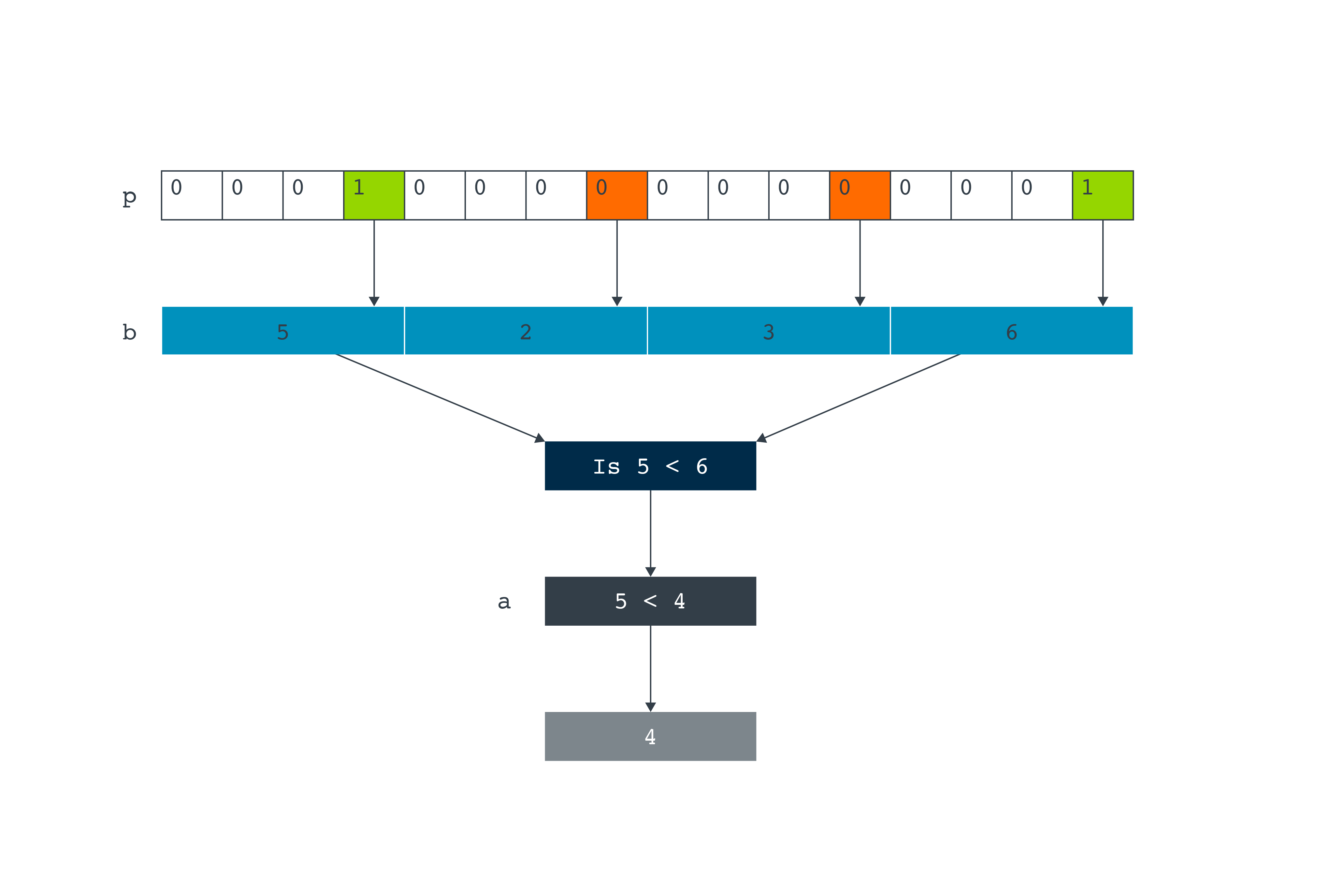## Predication

Predication provides a way to conditionally execute a block of instructions, on lanes that meet specified criteria. The predication mask specifies which lanes are processed by setting bits to true (1) or false (0).

Each bit represents the predication of a lane in the 128-bit Helium vector. Therefore, when using vectors which contain a lane width of 32 bits, 4 out of the 16 bits control predication. The following table shows different lane widths:

 Lane width Bits in `VPR.P0` 32 bits [12, 8, 4, 0] 16 bits [14, 12, 10, 8, 6, 4, 2, 0] 8 bits [15:0]

There are four different types of predication intrinsics:

`_m`
Merging
`_z`
Zeroing
`_x`
Don't care
`_p`
Predicated

The different types of predication can be used for loop tail handling. When input data is not a multiple of 128-bits, the final loop iteration needs to process a partially empty vector. For example, a data array of ten 32-bit values is processed as two full iterations on vectors containing four elements, and a final loop iteration on a vector containing two elements. Merging predication loads a value from the inactive vector into these lanes. Zeroing predication can mark the unused lanes on the final loop iteration. Pruning predication can only store the values in the lanes that are set to true. Don’t care predication can be used when we don’t care whether an undeclared or declared value are loaded into the unused lanes.

##### Examples of predication
Merging
Merging predication can be used for clipping values in a vector that exceed a specified maximum. Merging predication is where false predicated lanes, are filled with the corresponding element from the inactive vector.
The intrinsic `vaddq_m` is an example of a merging predication. `vaddq_m` adds two vectors together, and the false predicated lanes are filled with the value from the inactive vector. This is explained in the following example:
`int32x4_t [__arm_]vaddq_m[_s32] (int32x4_t inactive, int32x4_t a, int32x4_t b, mve_pred16_t p)`
In this example, the four inputs are:
• `Inactive`
• A 32x4 vector which contains 4,4,4,4
• `a`
• A 32x4 vector which contains 5,2,3,6
• `b`
• A 32x4 vector which contains 7, 1, 6, 2
• `p`
• A predicate mask, containing 16 bits. For 32-bit lanes, the bits in the mask which control lane predication are bits 12, 8, 4, and 0. In this example, bits 12 and 1 have been set to true and bits 8 and 4 have been set to false, resulting in a predicate mask of 0001000000000001.
The output vector is stored in`inactive`. This example uses a vector that is 32x4. The vector could be a different size. Helium registers has more information. However, the vector registers; inactive, a and b must contain the same number of lanes.
Looking at the lane predication in detail, we can see that:
• Bits 8 and 4 control lanes two and three. In our example, these bits contain zero. This means that lanes two and three take their value from the inactive vector. In both cases this value is four.
• Bits 12 and 0 control lanes one and four. In our example these bits contain one. This means that lanes one and four take their value from the result of adding the corresponding lanes in the vectors a and b.
• For bit 12 this corresponds to 5+7 = 12
• For bit 0 this corresponds to 6+8 = 14
Therefore, the result vector equals: 12,4,4,14.
This process is illustrated in the following diagram:The following assembly code assumes that r0 points to the `0x11111111 0x22222222 0x33333333 0x44444444` pattern in memory. The following code is an example of merging predication:
```// predicated addition (inactive lanes untouched)
mov             r1, 0xf00f      // set mask for 32-bit elements 0 & 3
vmsr            p0, r1          // set predicate bit 0 & 4
vldrw.s32       q0, [r0]        // q0 = { 0x11111111 0x22222222 0x33333333 0x44444444}
movw            r2, 0x5555
movt            r2, 0x5555
vdup.32         q1, r2          // q1 = {0x55555555 0x55555555 0x55555555 0x55555555}
vpst
vaddt.i32       q1, q0, q0      // q1 = {0x22222222 0x55555555 0x55555555 0x88888888}
```
```movw            r2, 0x0000      // set upper bound = 0x30000000
movt            r2, 0x3000
vldrw.s32       q0, [r0]        // q0 = { 0x11111111 0x22222222 0x33333333 0x44444444}
vpt.s32         ge, q0, r2      // enable lanes greater or equal than r2
vdupt.32        q0, r2          // set q0[i] to r2 for active lanes, others are untouched
// q0 = { 0x11111111 0x22222222 0x30000000 0x30000000}
```
Zeroing
Zeroing predication is used for load instructions. The false predicated lanes are set to zero.
The following intrinsic is an example of a zeroing predicated load, which loads consecutive elements from memory into a destination vector register:
`uint32x4_t [__arm_]vldrwq_z[_s32] (uint32_t const * base, mve_pred16_t p)`
Consider an example where the two inputs are:
• `Base`
• A pointer to the start of an array containing 32-bit unsigned integer values. In this example, it contains 5, 2, 3, 6.
• `p`
• A predicated mask. For 32-bit lanes, the bits in the mask which control lane predication are bits 12, 8, 4, 0. In this example, it equals 0000000000010001.
The output is a vector containing the result.,br> Looking at the lane predication in detail:
• Four numbers are contained within memory. These numbers are loaded into a vector through base, the pointer to the array.
• Bits 12 and 8 control lanes four and three. In our example, these bits contain zero. This means that lanes four and three are populated with the value zero.
• Bits 4 and 0 control lanes two and one. In our example, these bits contain one. This means that lanes one and two are populated with the value from memory.
Therefore, the result vector equals 0, 0, 2, 5.
This process is illustrated in the following diagram:The following assembly code assumes that `r0` points to the `0x11111111 0x22222222 0x33333333 0x44444444` pattern in memory. The following code is an example of zeroing predication:
```// starting with predicated load (zeroing of inactive lanes)
mov             r1, 0x0ff0      // set mask for 32-bit elements 1 & 2
vmsr            p0, r1          // set predicate bits
vpst                            // activate predication for the next slot
vldrwt.s32      q0, [r0]        // q0 = { 0x00000000 0x22222222 0x33333333
```
```// 8-bit load
mov             r1, 0x1111      // set mask for 8-bit elements 0, 4, 8, 12
vmsr            p0, r1          // set predicate bits
vpst                            // activate predication for the next slot
vldrbt.s8       q0, [r0]        // q0 = { 0x11 0x00 0x00 0x00 0x22 0x00 0x00 0x00 0x33 0x00 0x00 0x00 0x44 0x00 0x00 0x00}
```
```// 16-bit load
mov             r1, 0x300c      // set mask for 16-bit elements 1 & 6
vmsr            p0, r1          // set predicate bits
vpst                            // activate predication for the next slot
vldrht.s16      q0, [r0]        // q0 = { 0x0000 0x1111 0x000 0x00000 0x000 0x00000 0x4444 0x0000}
```
Don't care
Don’t care predication is like zeroing predication, because it is used for load instructions. The difference between don’t care predication and zeroing predication is that, when a lane has been set to false, an undeclared variable is left undefined instead of having a 0 as the output.
Predicated
Predicated predication is used when a scalar output is returned. False-predicated lanes are not used when computing the output.
The following intrinsic is an example of predicated predication. This intrinsic finds the minimum value of the elements in a vector, then compares that minimum value to the specified value a. The intrinsic returns the smaller of the two values:
`int32_t [__arm_]vminvq_p[_s32] (int32_t a, int32x4_t b, mve_pred16_t p)`
Consider an example in which the three inputs are:
• `a`
• A scalar value that equals 4
• `b`
• A 32x4 vector that contains the values: 5, 2, 3, 6
• `p`
• A predicated mask. For 32-bit lanes, the bits in the mask which control lane predication are bits 12, 8, 4, 0. In this example, it equals 0001000000000001
The output is the smaller of a or the minimum value in`b`.
Looking at the lane predication in detail:
• Bits 8 and 4 controls lanes two and three. In our example, these bits contain zero. This means that these lanes are not used.
• Bits 12 and 0 control lanes one and four. In our example, these bits contain one. This means that lanes one and four are compared to see which contains the smallest number. In this example, the smallest number is 5
• The minimum value: 5 is compared with the scalar value (`a`), which is 4.
• Therefore, the intrinsic returns 4.
This process is illustrated in the following diagram:The following assembly code assumes that `r0` points to the `0x11111111 0x22222222 0x33333333 0x44444444` pattern in memory. The following code is an example of predicated predication:
```// predicated 32-bit MAC (inactive lanes ignored)
mov             r1, 0x00ff      // set mask for 32-bit elements 0 & 1
vmsr            p0, r1          // set predicate bits
vldrw.s32       q0, [r0]        // q0 = { 0x11111111 0x22222222 0x33333333 0x44444444}
clrm            {r2, r3}
vpst
vrmlaldavht.s32  r2, r3, q0, q0 // r2,r3 = r2:r3 + (sum(q0[i] * q0[i]) + (1<<7)) >> 8         i={0,1}
//  (0x11111111 ^2 + 0x22222222 ^ 2  + (1<<7)) >> 8
```